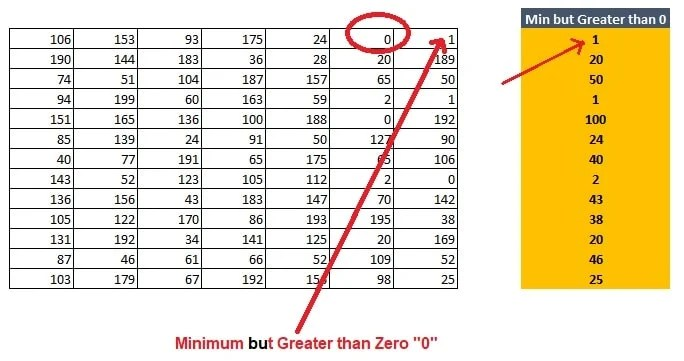# How to Calculate Minimum Value Greater than Zero “0” with Formula in Excel

Rate this post !

Calculate Minimum Value but Greater than Zero with Formula in ExcelFormula: {=MIN(IF(C2:I2>0,C2:I2))} (then Press Ctrl+Shft+Enter)

Video Tutorial